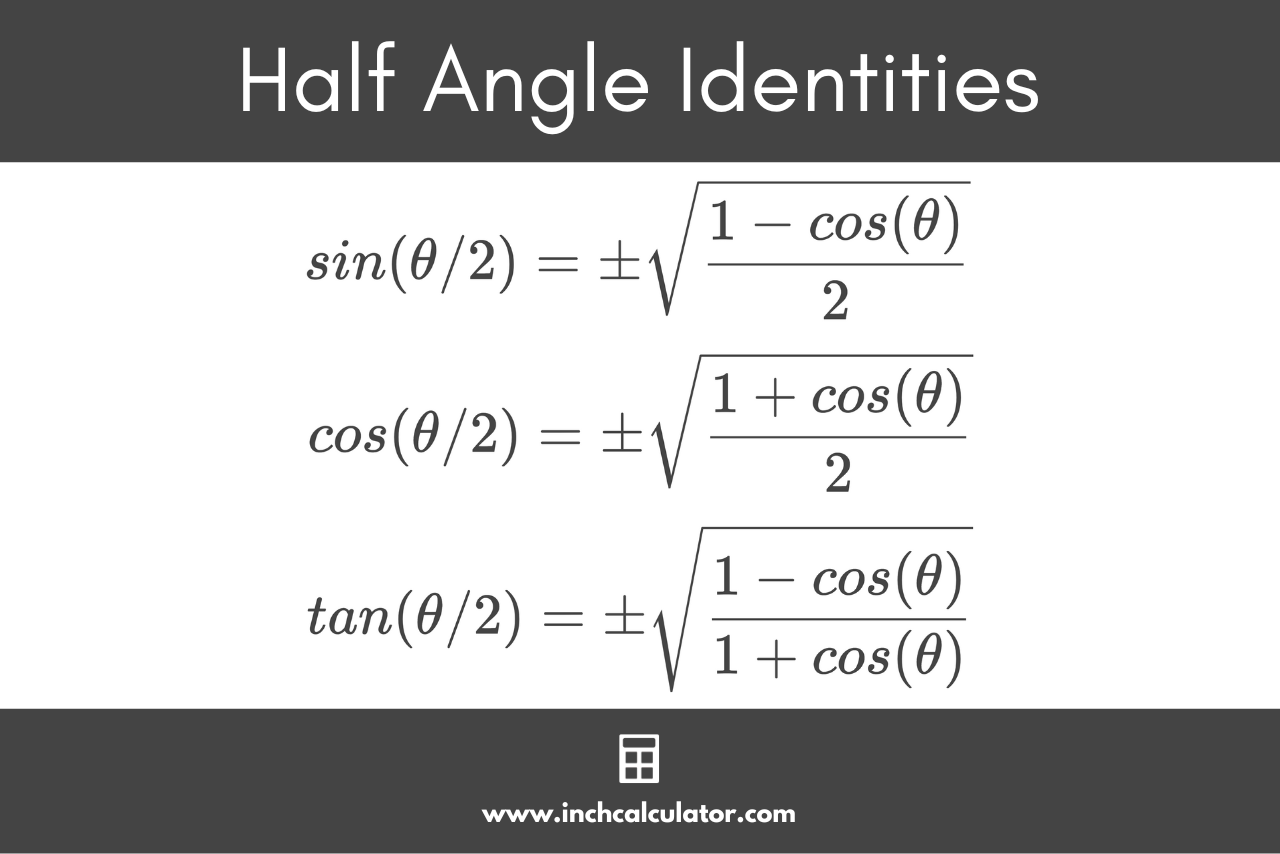# Half Angle Calculator

Use our half angle calculator to solve trigonometry problems with half angles. Insert the angle in degrees or radians below to calculate the result.

## Half-Angle Functions:

 sin(θ/2) = 0.64279 cos(θ/2) = 0.76604 tan(θ/2) = 0.8391
Learn how we calculated this below

## How to Solve Half Angles

Half angle identities express the trigonometric functions of half angles (denoted θ/2) in terms of the trigonometric functions of single angles θ. They are derived from the sum or difference of angle formulas, and are used to simplify complex expressions and prove trigonometric identities.

Calculating a half angle isn’t quite as easy as just dividing the result of the trigonometric function by 2.

There are six half angle identities, and just like double angles, there is a unique formula to express each one. See each formula below.### Sine Half Angle Formula

To calculate the sine of a half angle, you can use the following formula:

sin(\theta/2)=\pm\sqrt{\frac{1-cos(\theta)}{2}}

Thus, the sine of half an angle θ is equal to the square root of 1 minus the cosine of the angle θ divided by 2.

### Cosine Half Angle Formula

To calculate the cosine of a half angle, you can use the following formula:

cos(\theta/2)=\pm\sqrt{\frac{1+cos(\theta)}{2}}

Thus, the cosine of half an angle θ is equal to the square root of 1 plus the cosine of the angle θ divided by 2.

### Tangent Half Angle Formula

To calculate the tangent of a half angle, you can use the following formula:

tan(\theta/2)=\pm\sqrt{\frac{1- cos(\theta)}{1 + cos(\theta)}}

Thus, the tangent of half an angle θ is equal to the square root of 1 minus the cosine of the angle θ, divided by the square root of 1 plus the cosine of the angle θ.

### Cosecant Half Angle Formula

The cosecant of a half angle is equal to the reciprocal of the sine of the half angle. To calculate the cosecant of a half angle, you can use the following formula:

csc(\theta/2)=\pm\sqrt{\frac{2}{1-cos(\theta)}}

The cosecant of half an angle θ is equal to the square root of 2 divided by 1 minus the cosine of the angle θ.

### Secant Half Angle Formula

The secant of a half angle is equal to the reciprocal of the cosine of the half angle. To calculate the secant of a half angle, you can use the following formula:

csc(\theta/2)=\pm\sqrt{\frac{2}{1+cos(\theta)}}

The cosecant of half an angle θ is equal to the square root of 2 divided by 1 plus the cosine of the angle θ.

### Cotangent Half Angle Formula

The cotangent of a half angle is equal to the reciprocal of the tangent of the half angle. To calculate the cotangent of a half angle, you can use the following formula:

cot(\theta/2)=\pm\sqrt{\frac{1+cos(\theta)}{1-cos(\theta)}}

The cotangent of half an angle θ is equal to the square root of 1 plus the cosine of the angle θ, divided by the square root of 1 minus the cosine of the angle θ.